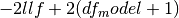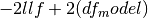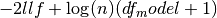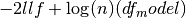# statsmodels.regression.linear_model.RegressionResults¶

class `statsmodels.regression.linear_model.``RegressionResults`(model, params, normalized_cov_params=None, scale=1.0, cov_type='nonrobust', cov_kwds=None, use_t=None)[source]

This class summarizes the fit of a linear regression model.

It handles the output of contrasts, estimates of covariance, etc.

Returns: Attributes aic Aikake’s information criteria. For a model with a constant. For a model without a constant. bic Bayes’ information criteria For a model with a constant. For a model without a constantbse The standard errors of the parameter estimates. pinv_wexog See specific model class docstring centered_tss The total (weighted) sum of squares centered about the mean. cov_HC0 Heteroscedasticity robust covariance matrix. See HC0_se below. cov_HC1 Heteroscedasticity robust covariance matrix. See HC1_se below. cov_HC2 Heteroscedasticity robust covariance matrix. See HC2_se below. cov_HC3 Heteroscedasticity robust covariance matrix. See HC3_se below. cov_type Parameter covariance estimator used for standard errors and t-stats df_model Model degress of freedom. The number of regressors p. Does not include the constant if one is present df_resid Residual degrees of freedom. n - p - 1, if a constant is present. n - p if a constant is not included. ess Explained sum of squares. If a constant is present, the centered total sum of squares minus the sum of squared residuals. If there is no constant, the uncentered total sum of squares is used. fvalue F-statistic of the fully specified model. Calculated as the mean squared error of the model divided by the mean squared error of the residuals. f_pvalue p-value of the F-statistic fittedvalues The predicted the values for the original (unwhitened) design. het_scale adjusted squared residuals for heteroscedasticity robust standard errors. Is only available after HC#_se or cov_HC# is called. See HC#_se for more information. HC0_se White’s (1980) heteroskedasticity robust standard errors. Defined as sqrt(diag(X.T X)^(-1)X.T diag(e_i^(2)) X(X.T X)^(-1) where e_i = resid[i] HC0_se is a cached property. When HC0_se or cov_HC0 is called the RegressionResults instance will then have another attribute het_scale, which is in this case is just resid**2. HC1_se MacKinnon and White’s (1985) alternative heteroskedasticity robust standard errors. Defined as sqrt(diag(n/(n-p)*HC_0) HC1_see is a cached property. When HC1_se or cov_HC1 is called the RegressionResults instance will then have another attribute het_scale, which is in this case is n/(n-p)*resid**2. HC2_se MacKinnon and White’s (1985) alternative heteroskedasticity robust standard errors. Defined as (X.T X)^(-1)X.T diag(e_i^(2)/(1-h_ii)) X(X.T X)^(-1) where h_ii = x_i(X.T X)^(-1)x_i.T HC2_see is a cached property. When HC2_se or cov_HC2 is called the RegressionResults instance will then have another attribute het_scale, which is in this case is resid^(2)/(1-h_ii). HC3_se MacKinnon and White’s (1985) alternative heteroskedasticity robust standard errors. Defined as (X.T X)^(-1)X.T diag(e_i^(2)/(1-h_ii)^(2)) X(X.T X)^(-1) where h_ii = x_i(X.T X)^(-1)x_i.T HC3_see is a cached property. When HC3_se or cov_HC3 is called the RegressionResults instance will then have another attribute het_scale, which is in this case is resid^(2)/(1-h_ii)^(2). model A pointer to the model instance that called fit() or results. mse_model Mean squared error the model. This is the explained sum of squares divided by the model degrees of freedom. mse_resid Mean squared error of the residuals. The sum of squared residuals divided by the residual degrees of freedom. mse_total Total mean squared error. Defined as the uncentered total sum of squares divided by n the number of observations. nobs Number of observations n. normalized_cov_params See specific model class docstring params The linear coefficients that minimize the least squares criterion. This is usually called Beta for the classical linear model. pvalues The two-tailed p values for the t-stats of the params. resid The residuals of the model. resid_pearson wresid normalized to have unit variance. rsquared R-squared of a model with an intercept. This is defined here as 1 - ssr/centered_tss if the constant is included in the model and 1 - ssr/uncentered_tss if the constant is omitted. rsquared_adj Adjusted R-squared. This is defined here as 1 - (nobs-1)/df_resid * (1-rsquared) if a constant is included and 1 - nobs/df_resid * (1-rsquared) if no constant is included. scale A scale factor for the covariance matrix. Default value is ssr/(n-p). Note that the square root of scale is often called the standard error of the regression. ssr Sum of squared (whitened) residuals. uncentered_tss Uncentered sum of squares. Sum of the squared values of the (whitened) endogenous response variable. wresid The residuals of the transformed/whitened regressand and regressor(s)

Methods

 `HC0_se`() See statsmodels.RegressionResults `HC1_se`() See statsmodels.RegressionResults `HC2_se`() See statsmodels.RegressionResults `HC3_se`() See statsmodels.RegressionResults `aic`() `bic`() `bse`() `centered_tss`() `compare_f_test`(restricted) use F test to test whether restricted model is correct `compare_lm_test`(restricted[, demean, use_lr]) Use Lagrange Multiplier test to test whether restricted model is correct `compare_lr_test`(restricted[, large_sample]) Likelihood ratio test to test whether restricted model is correct `condition_number`() Return condition number of exogenous matrix. `conf_int`([alpha, cols]) Returns the confidence interval of the fitted parameters. `cov_HC0`() See statsmodels.RegressionResults `cov_HC1`() See statsmodels.RegressionResults `cov_HC2`() See statsmodels.RegressionResults `cov_HC3`() See statsmodels.RegressionResults `eigenvals`() Return eigenvalues sorted in decreasing order. `ess`() `f_pvalue`() `fittedvalues`() `fvalue`() `get_robustcov_results`([cov_type, use_t]) create new results instance with robust covariance as default `mse_model`() `mse_resid`() `mse_total`() `nobs`() `resid`() `resid_pearson`() Residuals, normalized to have unit variance. `rsquared`() `rsquared_adj`() `scale`() `ssr`() `summary`([yname, xname, title, alpha]) Summarize the Regression Results `summary2`([yname, xname, title, alpha, ...]) Experimental summary function to summarize the regression results `uncentered_tss`() `wresid`()

Attributes

 `use_t`